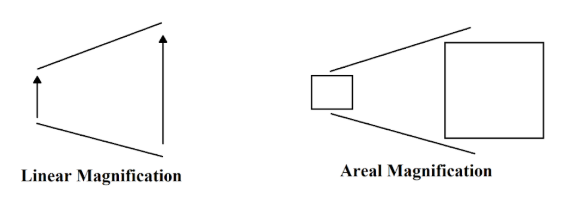QuestionAnswers

# A film projector magnifies a $100c{{m}^{2}}$ film strip on a screen. If the linear magnification is $4$, the area of the magnified film on the screen is $1000c{{m}^{2}}$?A. TrueB. FalseVerified
130.5k+ views
Hint: There can be two types of magnification associated with the image of an object. One is linear magnification, that considers the linear dimension of the object and the image and the other one is a real magnification that considers the areal dimensions of the object and the image. The ratio of the image to the area of the object is called Areal magnification. We will calculate the areal magnification on the film using the given linear magnification.

Formula used:
$\text{Areal magnification = }{{\left( \text{Linear magnification} \right)}^{2}}$

A projector is an optical instrument which is used to produce a large image of a small object. A film projector works on the principle of linear and areal magnification. The film is a long strip of translucent images which is positioned in the middle of a movie projector. Behind the film is a very bright lamp placed inside a concave reflector. White light from the lamp is concentrated through the lenses called as condenser on to the film. The filtered light, carrying the images on the film passes through a series of lenses that focuses the light onto the screen. When the light strikes the film, the image produced is many times larger than the original picture of the film.
Two types of Optical Magnification:
Linear Magnification – It considers the linear dimension of the object and the image.
Areal Magnification – It considers the areal dimensions of the object and the image.We are given that the film projector magnifies a $100c{{m}^{2}}$ film strip on the screen.
Linear magnification, $m=4$
Therefore,
Areal magnification,
\begin{align} & {{m}_{a}}={{m}^{2}}={{4}^{2}} \\ & {{m}_{a}}=16 \\ \end{align}
Surface area of film image on the screen is given as,
${{m}_{a}}\times 100=16\times 100=1600c{{m}^{2}}$
The area of the magnified film on the screen is $1600c{{m}^{2}}$

Hence, the correct option is B.

Note:
There are two conventional ways of specifying magnification, linear and areal magnification. $10\times$Linear magnification means that each dimension of the object appears ten times larger in the image whereas, $10\times$ areal magnification means that the apparent area of the magnified image is ten times the real area of the object.